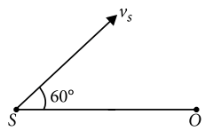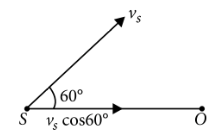Doppler
Question

# A source of sound S emitting waves of frequency 100 Hz and an observer O are located at some distance from each other. The source is moving with a speed of 19.4 $m{s}^{-1}$ at an angle of 60° with the source observer line as shown in the figure. The observer is at rest. The apparent frequency observed by the observer (velocity of sound in air 330 $m{s}^{-1}$, isModerate
Solution

## Here,As the velocity of source along the source observer line is ${v}_{s}$ cos 60° and the observer is at rest, so the apparent frequency observed by the observer is$v={v}_{0}\left(\frac{v}{v-{v}_{s}\mathrm{cos}60°}\right)$

Get Instant Solutions